# Class 10 NCERT Solutions- Chapter 2 Polynomials – Exercise 2.3

• Last Updated : 09 Mar, 2021

### Question 1. Divide the polynomial p(x) by the polynomial g(x) and find the quotient and remainder in each of the following:

(i) p(x) = x3 – 3x2 + 5x – 3, g(x) = x2 – 2

(ii) p(x) = x4 – 3x2 + 4x + 5, g(x) = x2 + 1 – x

Attention reader! All those who say programming isn't for kids, just haven't met the right mentors yet. Join the  Demo Class for First Step to Coding Coursespecifically designed for students of class 8 to 12.

The students will get to learn more about the world of programming in these free classes which will definitely help them in making a wise career choice in the future.

(iii) p(x) = x4– 5x + 6, g(x) = 2 – x2

Solution:

i) p(x) = x3 – 3x2 + 5x – 3, g(x) = x2 – 2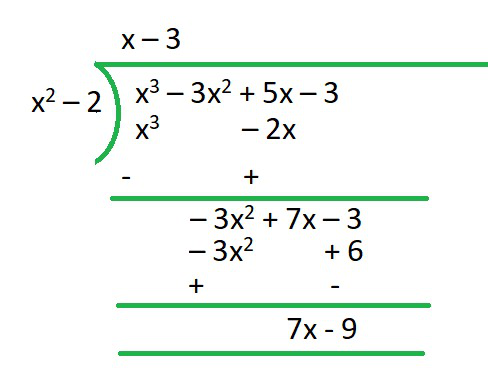R = 7x-9

Q = x-3

ii) p(x) = x4 – 3x2 + 4x + 5, g(x) = x2 + 1 – x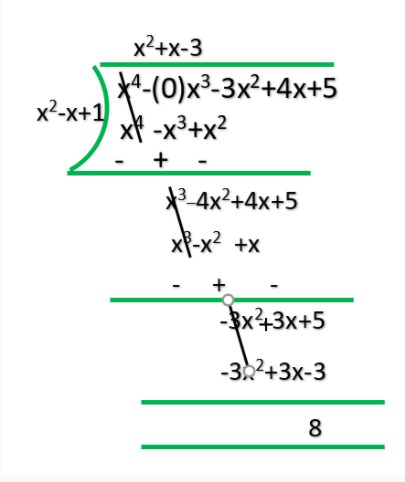R = 8

Q = x2+x-3

iii) p(x) = x4– 5x + 6, g(x) = 2 – x2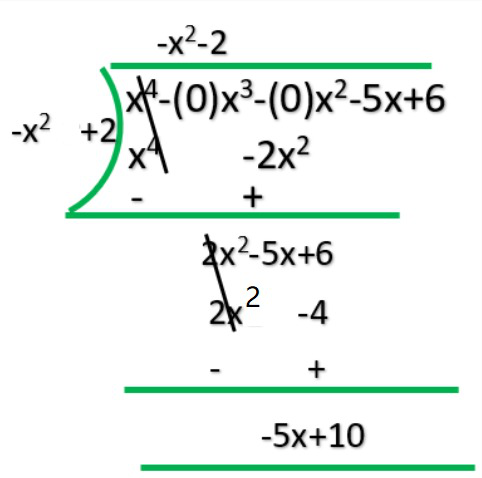Q = -x2-2

R = -5x+10

### Question 2. Check whether the first polynomial is a factor of the second polynomial by dividing the second polynomial by the first polynomial.

(i) t2 – 3, 2t4 + 3t3 – 2t2– 9t – 12

(ii) x2 + 3x + 1, 3x4 + 5x3 – 7x2 + 2x + 2

(iii) x3 – 3x + 1, x5 – 4x3 + x2 + 3x + 1

Solution:

i) t2 – 3, 2t4 + 3t3 – 2t2– 9t – 12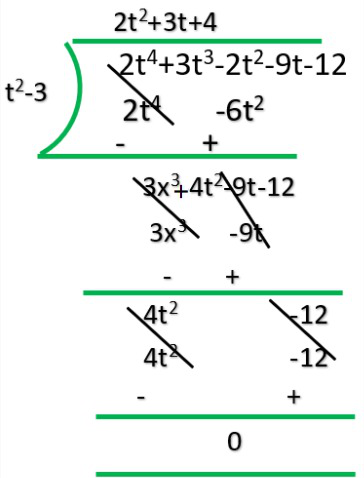Q = 2t3+3t+4

R = 0

Yes 1st polynomial is factor of 2nd polynomial.

ii) x2 + 3x + 1, 3x4 + 5x3 – 7x2 + 2x + 2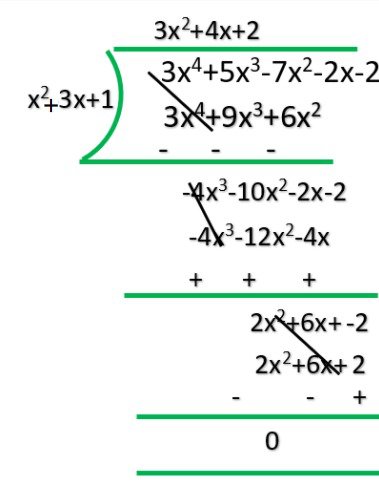R = 0

Q = 3x2-4x+2

iii) x3 – 3x + 1, x5 – 4x3 + x2 + 3x + 1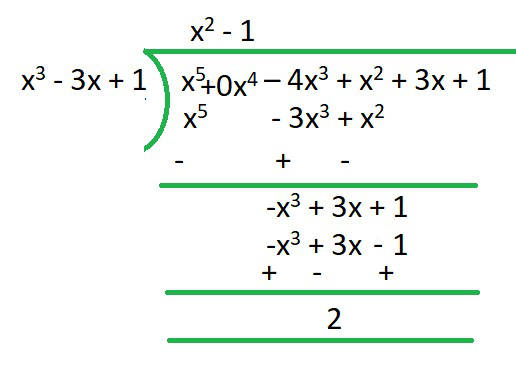R = x2-1

Q = 2

### Question 3. Obtain all other zeroes of 3x4 + 6x3 – 2x2 – 10x – 5, if two of its zeroes are and √(5/3) and -√(5/3).

Solution: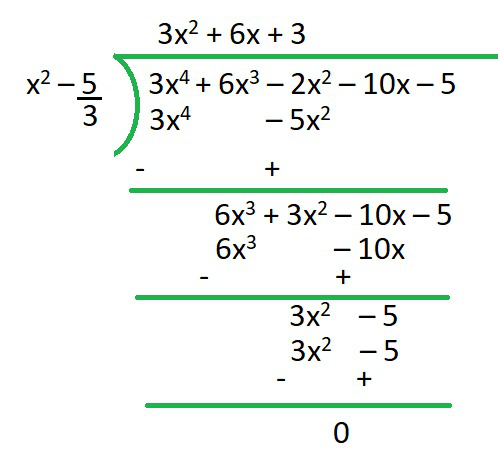R = 0

Q = 3x2+6x+3

∴ we are factorizing

3x2+6x+3

x2+2x+1

(x+1)2

(x+1)  (x+1) = 0

∴ x = -1 and x = -1### Question 4. On dividing x3 – 3x2 + x + 2 by a polynomial g(x), the quotient and remainder were x – 2 and -2x + 4 respectively. Find g(x).

Solution:

Dividend = Divisor * Quotient + Remainder

x3-3x2+3x-2/x-2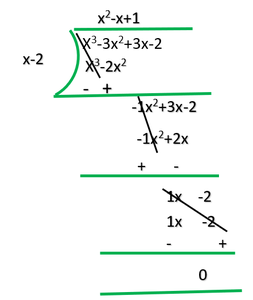R = 0

Q = x2 -x +1

### Question 5. Give examples of polynomials p(x), g(x), q(x) and r(x), which satisfy the division algorithm and:

(i) deg p(x) = deg q(x)

(ii) deg q(x) = deg r(x)

(iii) deg r(x) = 0

Solution:

i) deg p(x) = deg q(x)

p(x)=2x2-2x+14, g(x)=2

p(x)/g(x)=2x2-2x+14/2=(x2-x+7)

=x2-x+7=q(x)

=q(x)=x2-x+7

r(x)=0

ii) deg q(x)=deg r(x)

p(x)=4x2+4x+4, g(x)=x2+x+1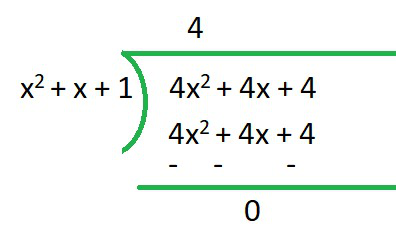q(x) = 4

r(x) = 0

∴Here deg q(x)=deg r(x)

iii) deg r(x)=0

p(x)=x3+2x2-x+2 ,g(x)=x2-1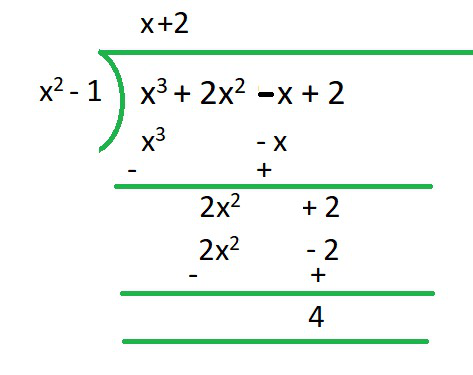q(x) = x+2

r(x) = 4

deg of r(x) = 0

My Personal Notes arrow_drop_up